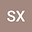$k$-sparse signal recovery via unrestricted $\ell_{1-2}$-minimization
•## Abstract

In the field of compressed sensing, $\ell_{1-2}$-minimization model can recover the sparse signal well. In dealing with the $\ell_{1-2}$-minimization problem, most of the existing literatures use the DCA algorithm to solve the unrestricted $\ell_{1-2}$-minimization model, i.e. model $(\ref{my1})$. Although experiments have proved that the unrestricted $\ell_{1-2}$-minimization model can recover the original sparse signal, the theoretical proof has not been established yet. This paper mainly proves theoretically that the unrestricted $\ell_{1-2}$-minimization model can recover the sparse signal well, and makes an experimental study on the parameter $\lambda$ in the unrestricted minimization model. The experimental results show that increasing the size of parameter $\lambda$ in model $(\ref{my1})$ appropriately can improve the recovery success rate. However, when $\lambda$ is sufficiently large, increasing $\lambda$ will not increase the recovery success rate.
12 May 2022Submitted to Electronics Letters
14 May 2022Submission Checks Completed
14 May 2022Assigned to Editor
20 May 2022Reviewer(s) Assigned
26 May 2022Review(s) Completed, Editorial Evaluation Pending
30 May 2022Editorial Decision: Revise Minor